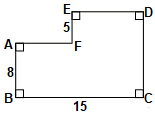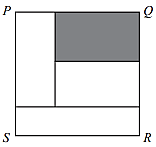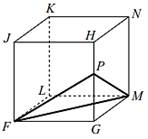CREST Mathematics Challenge Class 10 Sample Papers

REGISTER NOW

Syllabus:

The questions will be of three levels: Basic, Intermediate and Advanced from the following topics: Real Numbers, Polynomials, Pair of Linear Equations in Two Variables, Quadratic Equations, Arithmetic Progressions, Triangles, Coordinate Geometry, Introduction to Trigonometry, Some Applications of Trigonometry, Circles, Constructions, Areas Related to Circles, Surface Areas and Volumes, Statistics, Probability.

 Q.1 In a jar, the ratio of the number of dimes to the number of quarters is 3 : 2. If the total value of these coins is \$4, how many dimes are in the jar? (Each dime is worth 10 cents, each quarter is worth 25 cents, and \$1 equals 100 cents.)
 Q.2 How many different lists a, b, c, d of distinct odd positive integers with a < b < c < d have the property that a + b + c + d = 24?
 Q.3 Figure ABCDEF has AB = 8, BC = 15, and EF = 5, as shown. Determine the perimeter of ABCDEF.Q.4 The line with equation y = 2x − 6 is reflected in the y-axis. What is the x-intercept of the resulting line?
 Q.5 There are three distinct real numbers a, b and c that are solutions of the equation x3 − 4x = 0. What is the value of the product abc?
 Q.6 In the diagram, square P QRS has side length 42 and is divided into four non-overlapping rectangles. If each of these four rectangles has the same perimeter, what is the area of the shaded rectangle?Q.7 A cube with vertices FGHJKLMN has edge length 200. Point P is on HG, as shown. The shortest distance from G to a point inside 4P FM is 100. Which of the following is closest to the length of HP?Q.8 Paul has 6 boxes, each of which contains 12 trays. Paul also has 4 extra trays. If each tray can hold 8 apples, what is the largest possible number of apples that can be held by the 6 boxes and 4 extra trays?
 Q.9 A container in the shape of a triangular prism stands on one of its triangular faces. Three spheres of radius 1 are placed inside the container, each touching the triangular bottom. Each sphere touches two of the rectangular faces of the container and each sphere touches the other two spheres. A fourth sphere of radius 1 is placed on top of the three spheres, touching each of the three spheres and the top of the prism. The volume of the prism is closest to:
 Q.10 A die is a cube with its faces numbered 1 through 6. One red die and one blue die are rolled. The sum of the numbers on the top two faces is determined. What is the probability that this sum is a perfect square?

Sample PDF of CREST Mathematics Challenge for Class 10: